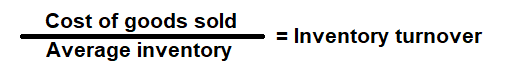# Inventory Turnover Ratio Calculator

Key in theCost of goods Solds (COGS) and Inventory Values to the respective fields given below and then click Calculate to get the desired result.

# Inventory Turnover Ratio Calculator - Glossary:

Inventory Turnover Ratio: Shows the proportion of Cost of Goods Sold (COGS) to Average Inventory. In simple words, is a key measure for evaluating how efficient the business is at managing its inventory and generating sales.

### Formula:### How to use this equation?

The values are commonly stated against inventory in the balance sheet and net sales in the income statement. To use this ratio, divide cost of goods sold by its average inventory.
Note:
Average Inventory: Add inventory values of the current (ending) year and the inventory values of the previous (beginning) year, and then divide that value by 2.

Cost of Goods Sold (COGS):
Cost of goods sold (COGS) is total cost to produce goods and services such as cost of materials, wages and other expenses related to producing a goods and service.

Inventory:
Inventory is the finished goods that can be sold immediately.

Example:
A company with a cost of goods (COGS) of \$225,000, beginning inventory of \$20,000 and ending inventory of \$25,000 will have inventory turnover ratio of 10 times. It means that the company sold its entire inventory within 36.5 days. This metric helps a company to determine how long it will take to sell and replace its inventory and can help plan its inventory level or re-orders.